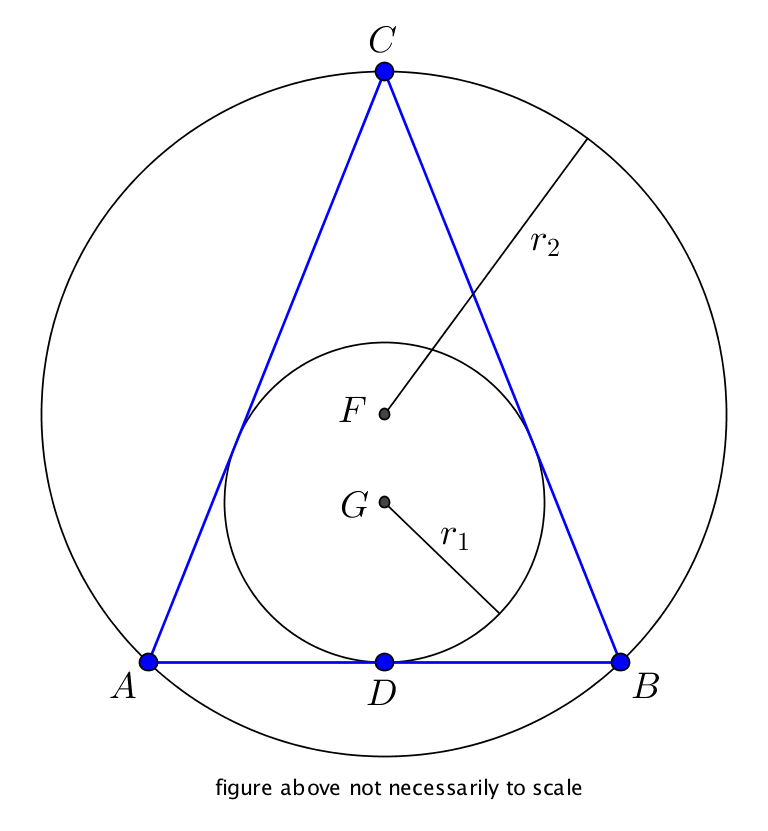# Tricky altitudes

Geometry Level 5

$\Delta ABC$ is an isosceles triangle with $AC = BC$ and $AB = 2$. $D$ is the midpoint of $AB$. The ratio of the radii of it's inscribed circle (radius is $r_1$) and circumscribed circle (radius is $r_2$) is $3:8$.Two different triangles can be formed under these conditions. In one triangle the square of length $CD$ is an integer $a$, in the other the square of length $CD$ can be expressed as $\frac{m}{n}$, where $m$ and $n$ are coprime positive integers.

What is the value of $a + m + n$?

×